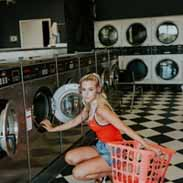### We've found 13 Balanced Chemical Equation tests

Balanced Chemical Equation Equations Formulas Hydrogen And Oxygen
Chemistry Ch. 11 Key Concepts (Prentice Hall) – Flashcards 23 termsLewis Gardner
23 terms
Balanced Chemical Equation Chemistry
Ch 15 Chemical Equillibrium – Flashcards 210 termsMatilda Campbell
210 terms
Balanced Chemical Equation Chemistry General Chemistry
chemistry chapter 10 study guide – Flashcards 20 termsMya Day
20 terms
Balanced Chemical Equation Chemistry Temperature And Pressure
Chemistry Matter and Change: Chapter 11 Stoichiometry – Flashcards 33 termsJay Barber
33 terms
Balanced Chemical Equation Chemical Engineering Chemistry Conservation Of Mass Left Hand Side
Chemistry: Semester 1 Final Exam Study Guide – Flashcards 33 termsKieran Carr
33 terms
Atoms Balanced Chemical Equation Chemistry Ratios
Chemistry Semester 2 Final Exam Study Guide – Flashcards 59 termsLewis Gardner
59 terms
AP Chemistry Balanced Chemical Equation Chemistry Mouth And Throat Pharmaceutical Sciences The Ear
Dermatology Therapies – Flashcards 134 termsMarguerite Castillo
134 terms
Balanced Chemical Equation Chemical Engineering Chemistry
Chapter 12 Stoichiometry – Flashcards 15 termsKen Ericksen
15 terms
Balanced Chemical Equation Chemical Engineering Chemistry
Chapter 4 notes – Flashcards 24 termsJudith Simpson
24 terms
AP Chemistry Balanced Chemical Equation Chemistry Equilibrium Lower Case Letters Physical Chemistry
Chem 106 – Chemistry – Flashcards 90 terms90 terms
Balanced Chemical Equation Geology Groundwater
CHM Final UCF – Flashcards 55 terms55 terms
Balanced Chemical Equation Chemistry Physical Chemistry Specific Heat Capacity
Final Review Ch. 11 (math) – Flashcards 8 termsRuth Blanco
8 terms
AP Biology Atoms Balanced Chemical Equation Biology Chemistry GCSE Chemistry
Final Review Chemistry HRA – Flashcards 73 termsJose Escobar
73 terms
Write a balanced chemical equation for the reaction that occurs when the hydrocarbon styrene, C8H8(l), is combusted in air.C8H8(l)+10O2(g)→8CO2(g)+4H2O(l)
Write a balanced chemical equation for the reaction that occurs when heptane, C7H16(l) , burns in air.C7H16(l)+11O2(g)→7CO2(g)+8H2O(g)
Write a balanced chemical equation for the reaction that occurs when the gasoline additive methyl tert-butyl ether, C5H12O(l), burns in air.2C5H12O(l)+15O2(g)→10CO2(g)+12H2O(g)
In terms of what quantities can you interpret a balanced chemical equation?a balanced chemical equation can be interpreted in terms of different quantities, including numbers of atoms, molecules, or moles; mass; and volume.
Write a balanced chemical equation for the reaction that occurs when calcium metal undergoes a combination reaction with O2(g).Write a balanced chemical equation for the reaction that occurs when Mg(s) reacts with Cl2(g).Mg(s)+Cl2(g)→MgCl2(s)
Write a balanced chemical equation for the reaction that occurs when barium carbonate decomposes into barium oxide and carbon dioxide gas when heated.Write a balanced chemical equation for the reaction that occurs when copper (II) hydroxide decomposes into copper (II) oxide and water when heated.How does a chemist use balanced chemical equation?The unbalanced chemical equation for the reaction in which carbon monoxide burns in oxygen to form carbon dioxide is?